# Current, Charge & Potential Difference

A. Charge

Matter is made of tiny particles called atoms. An atom consists of protons, neutrons and electrons. Normally, the number of protons and electrons are equal and the overall charge of an atom is zero. But, for certain atoms, electrons in the outer shell of an atom can be easily removed. An atom with such unbalanced positive charges becomes positively charged and is called a positive ion. Electric charge is measured in coulombs (C) and an electron consists of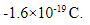B. Current

Atoms in metals have one or more free electrons, which are not tightly held to the nucleus. These free electrons move randomly throughout the metal. When a battery is connected to the metal wire, the electrons are repelled from the negative terminal of the battery and are attracted towards the positive terminal. These flows of electrons produce electric current. Current is measured in amperes (A).

A current of 4 amperes means that 4 coulombs of charge pass a point in the circuit every second. In 3 seconds, a total charge of 12 coulombs will have passed the point. If the amount of charge (Q) passes through the circuit for time (t), current (I) flows through it. This can be mathematically represented asWhen the current is changing over time, the amount of charge is calculated using the current-time graph.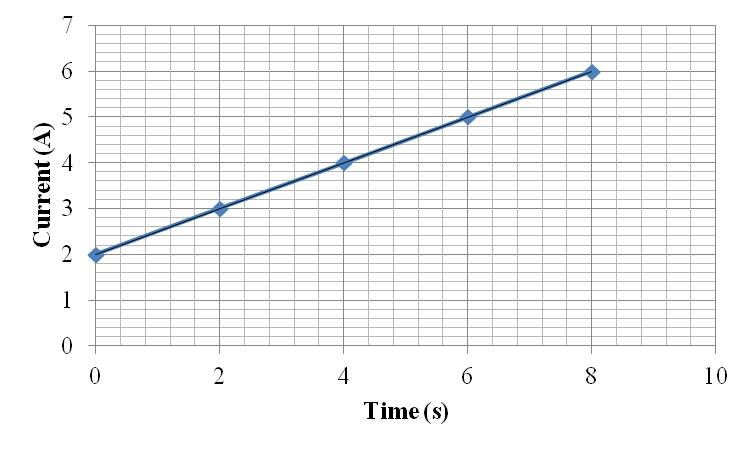Figure 1: Current-time Graph

Let us consider the current-time graph given in Figure 1. The total amount of charge flowing through the circuit can be found by measuring the area under the graph.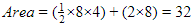32 coulombs of charge passes through the circuit in 8 seconds.

Figure 2 shows an electric circuit that consists of a battery, wire, switch and bulb. The wire and metal filament of the bulb is made of metal. Atoms of a metal have one or more electrons that are free to move. These electrons are called free electrons.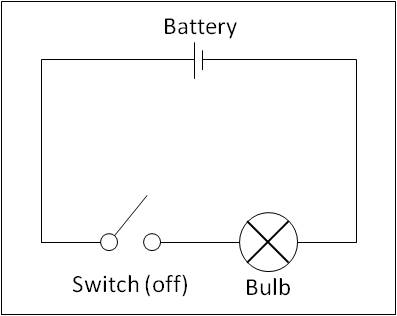Figure 2: Simple Electric Circuit

When the switch is closed, the electrons move from the negative terminal to the positive terminal due to the energy from the battery and the bulb glows. This flow of electrons is called a current. Benjamin Franklin thought electricity flows from a positively charged substance to a negatively charged substance. His idea is still used today and is known as the conventional current direction. Figure 3 shows the direction of electron flow and conventional current direction.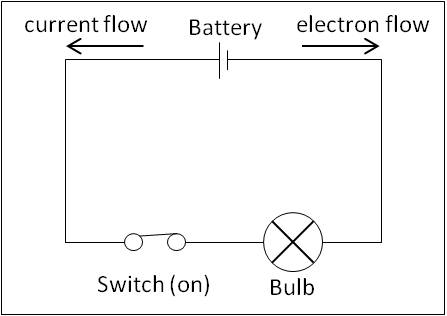Figure 3: Conventional Current Flow

C. Conduction in a current carrying conductor

Let us consider a part of a conductor as shown in the figure below. The cross-sectional area of the conductor is A. The movement of positive and negative charges is shown. Positive charges move towards the left and the negative charges move towards the right. The current flowing through the conductor is also shown. The direction of the current is in the direction of movement of positive charges. The drift speed of the charges is v.Figure 4: Charges Flowing Through the Conductor

The flow of charges is characterised by an average drift speed. The average drift speed of the charges is v. The number density (the number of charges per unit volume) is n. The charge on the current carriers is q.

The number of charges in length (x) of a conductor is nAx. The amount of positive charge that leaves this volume through the left-hand side of the conductor in time (t) is nAxq. The time interval t is x/v.

Hence, current can be given asD. Potential Difference

In Figure 1, the electrons obtain energy from the battery to flow through the circuit. A potential difference is set up across the positive and negative terminals of the battery, due to which the charges flow.

The potential difference between any two points in a circuit is the measure of work done by an electron to move from one point to another. Potential energy is measured in volts (V) and energy is measured in joules (J).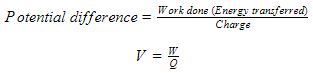One volt is the potential difference between two points, when one joule of energy is transferred from electrical to other forms by one coulomb of electric charge passing from one point to other.

E. Measuring Current

Ammeters are used to measure current flowing through the circuit at a specific point. The ammeter is connected in series to the point as shown in the figure below.Figure 5: Measuring Current

F. Measuring Potential Difference

Voltmeters are used to measure the potential difference between two points. Hence, it is connected in parallel between the two points where the potential difference needs to be measured, as shown in the figure below.Figure 6: Measuring Potential Difference

D. Summary

• Electric current is the rate of flow of a charge: I=Q/t.
• Conventional current is a flow of charges from positive to negative terminals.
• The coulomb is the unit of charge and is equivalent to an ampere-second.
• Charges can be calculated using the equation Q=It.
• For a current-carrying conductor, I=nAvq.
• The potential difference between any two points in a circuit is the measure of work done by an electron to move from one point to another, V=W/q.
• Current is measured using an ammeter and is placed in series to the component.
• Potential difference is measured using a voltmeter and is placed across the component.